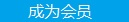您可以捐助，支持我们的公益事业。 1元 10元 50元 认证码：必填求知 文章 文库 Lib 视频 iProcess 课程 认证 咨询 工具 讲座 Modeler Code要资料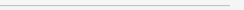订阅捐助
Python机器学习项目实战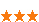1410 次浏览     评价： 好 中 差
2018-10-23

 编辑推荐: 本文来自于cloud.tencent.com，作者以浅显易懂的语言和清晰的示例和代码教你从头开始走过一个机器学习之旅，并且附详细的代码，大家可以收藏和学习。――Putting the machine learning pieces together

1. 数据清理和格式化

2. 探索性数据分析

3. 特征工程和特征选择

4. 在性能指标上比较几种机器学习模型

5. 对最佳模型执行超参数调整

6. 在测试集合中评估最佳模型

7. 解释模型结果

8. 得出结论

GitHub完整项目链接

 import pandas as pd import numpy as np # Read in data into a dataframe data = pd.read_csv('data/Energy_and_Water_Data_Disclosure_for_ Local_Law_84_2017__Data_for_Calendar_Year_2016_.csv') # Display top of dataframe data.head()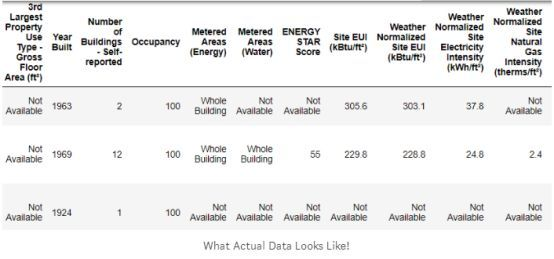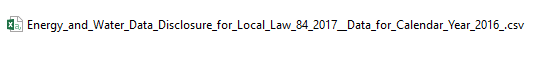# See the column data types and non-missing values data.info()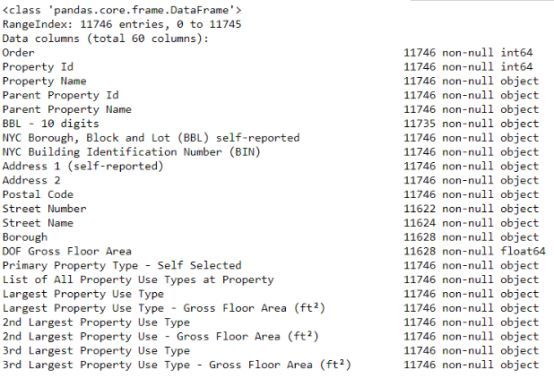# Replace all occurrences of Not Available with numpy not a number data = data.replace({'Not Available': np.nan}) # Iterate through the columns for col in list(data.columns): # Select columns that should be numeric if ('ft2' in col or 'kBtu' in col or 'Metric Tons CO2e' in col or 'kWh' in col or 'therms' in col or 'gal' in col or 'Score' in col): # Convert the data type to float data[col] = data[col].astype(float)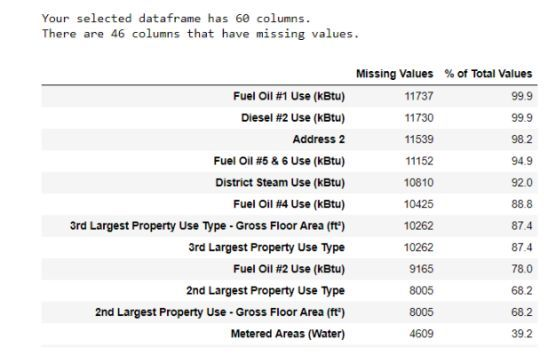（为了创建这个表，我使用了这个Stack Overflow论坛的一个函数【6】）。

1. the first quartile ? 3 ? interquartile range

2. he third quartile + 3 ? interquartile range

（有关删除列和异常的代码，请参阅notebook）。 在数据清理和异常清除过程结束时，我们剩下11,000多个建筑物和49个特征。

 import matplotlib.pyplot as plt # Histogram of the Energy Star Score plt.style.use('fivethirtyeight') plt.hist(data['score'].dropna(), bins = 100, edgecolor = 'k'); plt.xlabel('Score'); plt.ylabel('Number of Buildings'); plt.title('Energy Star Score Distribution');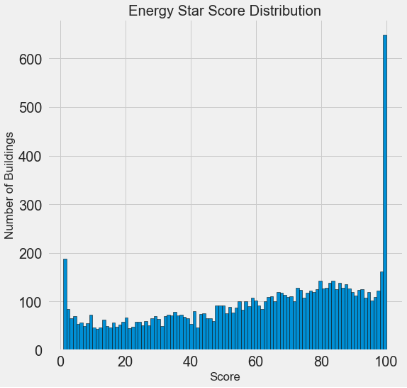EDA的主要部分是搜索特征和目标之间的关系。与目标相关的变量对模型很有用，因为它们可用于预测目标。通过使用seaborn库的密度图可以检查目标上的分类变量（仅采用有限的一组值）的效果。

 # Create a list of buildings with more than 100 measurements types = data.dropna(subset=['score']) types = types['Largest Property Use Type'].value_counts() types = list(types[types.values > 100].index) # Plot of distribution of scores for building categories figsize(12, 10) # Plot each building for b_type in types: # Select the building type subset = data[data['Largest Property Use Type'] == b_type] # Density plot of Energy Star scores sns.kdeplot(subset['score'].dropna(), label = b_type, shade = False, alpha = 0.8); # label the plot plt.xlabel('Energy Star Score', size = 20); plt.ylabel('Density', size = 20); plt.title('Density Plot of Energy Star Scores by Building Type', size = 28);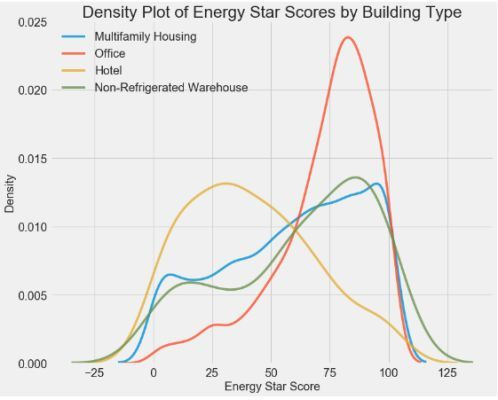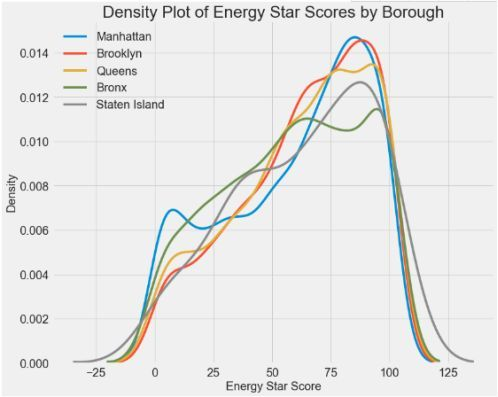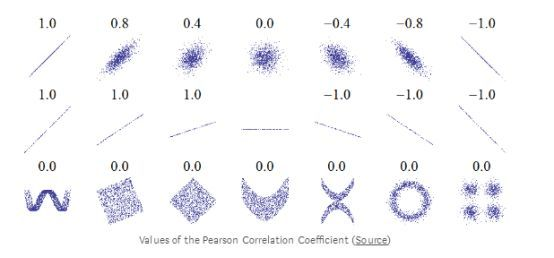# Find all correlations with the score and sort correlations_data = data.corr()['score'].sort_values()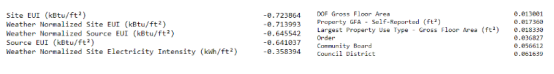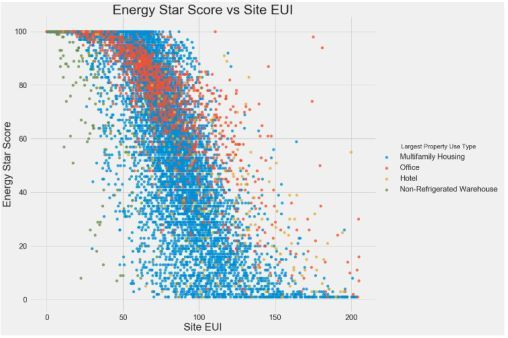# Extract the columns to plot plot_data = features[['score', 'Site EUI (kBtu/ft2)', 'Weather Normalized Source EUI (kBtu/ft2)', 'log_Total GHG Emissions (Metric Tons CO2e)']] # Replace the inf with nan plot_data = plot_data.replace({np.inf: np.nan, -np.inf: np.nan}) # Rename columns plot_data = plot_data.rename(columns = {'Site EUI (kBtu/ft2)': 'Site EUI', 'Weather Normalized Source EUI (kBtu/ft2)': 'Weather Norm EUI', 'log_Total GHG Emissions (Metric Tons CO2e)': 'log GHG Emissions'}) # Drop na values plot_data = plot_data.dropna() # Function to calculate correlation coefficient between two columns def corr_func(x, y, **kwargs): r = np.corrcoef(x, y) ax = plt.gca() ax.annotate("r = {:.2f}".format(r), xy=(.2, .8), xycoords=ax.transAxes, size = 20) # Create the pairgrid object grid = sns.PairGrid(data = plot_data, size = 3) # Upper is a scatter plot grid.map_upper(plt.scatter, color = 'red', alpha = 0.6) # Diagonal is a histogram grid.map_diag(plt.hist, color = 'red', edgecolor = 'black') # Bottom is correlation and density plot grid.map_lower(corr_func); grid.map_lower(sns.kdeplot, cmap = plt.cm.Reds) # Title for entire plot plt.suptitle('Pairs Plot of Energy Data', size = 36, y = 1.02);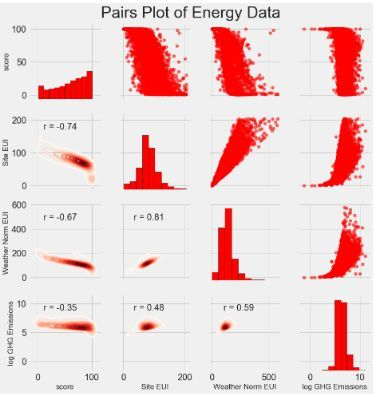One-hot编码分类变量（borough and property use type）。

 # Copy the original data features = data.copy() # Select the numeric columns numeric_subset = data.select_dtypes('number') # Create columns with log of numeric columns for col in numeric_subset.columns: # Skip the Energy Star Score column if col == 'score': next else: numeric_subset['log_' + col] = np.log(numeric_subset[col]) # Select the categorical columns categorical_subset = data[['Borough', 'Largest Property Use Type']] # One hot encode categorical_subset = pd.get_dummies(categorical_subset) # Join the two dataframes using concat # Make sure to use axis = 1 to perform a column bind features = pd.concat([numeric_subset, categorical_subset], axis = 1)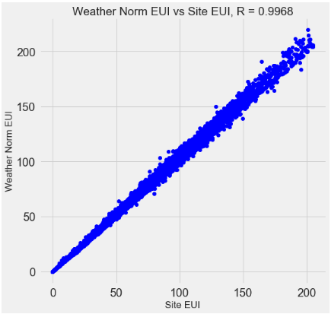# Remove any columns with all na values features = features.dropna(axis=1, how = 'all') print(features.shape) (11319, 65)

1. 训练集是我们在训练期间给我们的模型提供特征以及答案的。目地是让模型学习特征与目标之间的映射。

2. 测试集合的特征用于评估训练的模型。模型不允许查看测试集的答案，并且只能使用特征进行预测。我们知道测试集的答案，因此我们可以将测试预测与答案进行比较。

 # Split into 70% training and 30% testing set X, X_test, y, y_test = train_test_split(features, targets, test_size = 0.3, random_state = 42)

 # Function to calculate mean absolute error def mae(y_true, y_pred): return np.mean(abs(y_true - y_pred)) baseline_guess = np.median(y) print('The baseline guess is a score of %0.2f' % baseline_guess) print("Baseline Performance on the test set: MAE = %0.4f" % mae(y_test, baseline_guess))

 The baseline guess is a score of 66.00 Baseline Performance on the test set: MAE = 24.5164

Baseline的估计在测试集中约为25分。 得分范围从1到100，所以这代表25％的误差，相当低的一个超越！

1. 清理并格式化原始数据

2. 进行探索性数据分析以了解数据集

3. 开发了一系列我们将用于模型的特征1410 次浏览  评价: 好 中 差订阅捐助
 相关文章 我们该如何设计数据库 数据库设计经验谈 数据库设计过程 数据库编程总结
 相关文档 数据库性能调优技巧 数据库性能调整 数据库性能优化讲座 数据库系统性能调优系列
 相关课程 高性能数据库设计与优化 高级数据库架构师 数据仓库和数据挖掘技术 Hadoop原理、部署与性能调优每天2个文档/视频 扫描微信二维码订阅
 订阅技术月刊 获得每月300个技术资源